`import numpy as npimport pandas as pdimport matplotlib.pyplot as pltimport seaborn as snstrain_data = pd.read_csv("data/train_data.csv", )`
• user: a unique ID assigned to each user
• display: an ID related to the frame on which this ad was being shown
• widgetId: the place of the ad’s frame on the display
• device: user’s device model
• OS: user’s operating system
• Browser: user’s browser
• doc: web page’s ID
• source: website’s ID
• clicked: the actual target value (1 means that the user has clicked on the ad. Otherwise, it is 0)
`# Doing the upsamplingclicked_samples = train_data[train_data['clicked'] == 1]non_clicked_samples = train_data[train_data['clicked'] == 0]clicked_samples = clicked_samples.sample(replace=True, n=len(non_clicked_samples), random_state=1) # upsamplingtrain_data = pd.concat([clicked_samples, non_clicked_samples])`
`sr = train_data.isna().sum() # there is no null data in trainingtrain_data['bias'] = np.ones(train_data.shape) # add bias termtrain_data = train_data.sample(frac=1) # shuffle data`
`correlation_matrix = train_data.corr().round(2)sns.heatmap(data=correlation_matrix, annot=False)plt.show()`
`# Features which we won't usetrain_data = train_data.drop(['displayId', 'timestamp', 'docId', 'userId'], axis=1)# Features which we will use -- doing one hot encodingfeatures = ['dayOfWeek', 'hourOfDay', 'advertiserId', 'campaignId', 'creativeId', 'publisher', 'widgetId', 'device', 'os', 'browser', 'source']`
`from scipy.sparse import *one_hot = pd.get_dummies(train_data, columns=features, sparse=True)one_hot.shapeone = one_hot.astype('Sparse')matrix = one.sparse.to_coo().tocsr() # Converting to SciPy sparse matrix`
`matrix_X = matrix[:, 1:] # Xmatrix_y = matrix[:, 0] # Y`
`from sklearn.model_selection import train_test_splitX_train, X_test, y_train, y_test = train_test_split(matrix_X, np.squeeze(np.asarray(matrix_y.todense())), test_size=0.2) # This funcion would work efficiently.X_train, X_valid, y_train, y_valid = train_test_split(X_train, y_train, test_size=0.25) # 0.25 * 0.8 = 0.2`
`from sklearn.metrics import *from sklearn.ensemble import RandomForestClassifierclf = RandomForestClassifier(max_depth=4, random_state=0, n_estimators=80)clf.fit(X_train, y_train)print("end training")preds = clf.predict(X_test)preds_proba = clf.predict_proba(X_test)print("F1:           ", f1_score(y_test, preds))fpr, tpr, thresholds = roc_curve(y_test, preds_proba[ : , 1], pos_label=None)print("AUC:          ", auc(fpr, tpr))print("Cross Entropy:", log_loss(y_test, preds_proba))print("Accuracy:     ", accuracy_score(y_test, preds))`
`end training F1:            0.6087770079244672 AUC:           0.6028115864061809 Cross Entropy: 0.6893415826593268 Accuracy:      0.5632055069625873`
`from sklearn.svm import SVCclf = SVC(C=1)clf.fit(X_train.head(1000), y_train.head(1000))print("end training")preds = clf.predict(X_test)preds_proba = clf.predict_proba(X_test)print("F1:           ", f1_score(y_test, preds))fpr, tpr, thresholds = roc_curve(y_test, preds, pos_label=None)print("AUC:          ", auc(fpr, tpr))`
`end training F1:            0.6057987208137474 AUC:           0.5338544225761602 `
`from sklearn.linear_model import LogisticRegressionclf = LogisticRegression(random_state=0, C=1, max_iter=1200)clf.fit(X_train, y_train)print("end training")preds = clf.predict(X_test)preds_proba = clf.predict_proba(X_test)print("F1:           ", f1_score(y_test, preds))fpr, tpr, thresholds = roc_curve(y_test, preds, pos_label=None)print("AUC:          ", auc(fpr, tpr))print("Cross Entropy:", log_loss(y_test, preds_proba))`
`end training F1:            0.6244800254253621 AUC:           0.6204446745676685 Cross Entropy: 0.6487833714971616`
`import xgboost as xgbclf = xgb.XGBClassifier(max_depth=4,  n_estimators=80)clf.fit(X_train, y_train)print("end training")preds = clf.predict(X_test)preds_proba = clf.predict_proba(X_test)print("F1:           ", f1_score(y_test, preds))fpr, tpr, thresholds = roc_curve(y_test, preds, pos_label=None)print("AUC:          ", auc(fpr, tpr))print("Cross Entropy:", log_loss(y_test, preds_proba))`
`end training F1:            0.6058749030148753 AUC:           0.6267827426157575 Cross Entropy: 0.6713597341509303`
`from sklearn.datasets import dump_svmlight_filedump_svmlight_file(X_train, y_train, 'data/FM_train.libsvm')dump_svmlight_file(X_test, y_test, 'data/FM_test.libsvm')`
`# !pip install xlearnimport xlearn as xlfm_model = xl.create_fm()fm_model.setTrain("data)/FM_train.libsvm")param = {'task':'binary', 'lr':0.2,'lambda':0.002, 'metric':'acc'}fm_model.fit(param, 'data/model.out')fm_model.setTest('data/FM_test.libsvm')  # Test datafm_model.setSigmoid()  # Convert output to 0-1# Start to predict# The output result will be stored in output.txtfm_model.predict("data/model.out", "data/output.txt")`
`AUC:           0.6463157655406329Cross Entropy: 0.675787874373196`

--

--

--

## More from Ashkan Mirzaei

Love podcasts or audiobooks? Learn on the go with our new app.

## Geometric meaning of a trace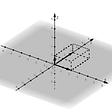## 3 Highlights from FAT*ML 2020## Dimensionality reduction: an easy approach to PCA and TSNE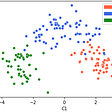## Explained: Deep Learning in Tensorflow — Chapter 0## Your Deep-Learning-Tools-for-Enterprises Startup Will Fail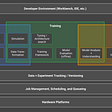## Q means Algorithm | Quantum Machine Learning 102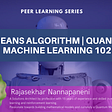## Semantic Search : Driven By AI## Understanding ConvMixer (with a simple PyTorch implementation)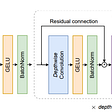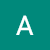## Accuracy — Performance prediction of the model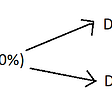## The Missing Library in your Machine Learning Workflow## Feature scaling and dummy encoding, and their application in Scikit Learn